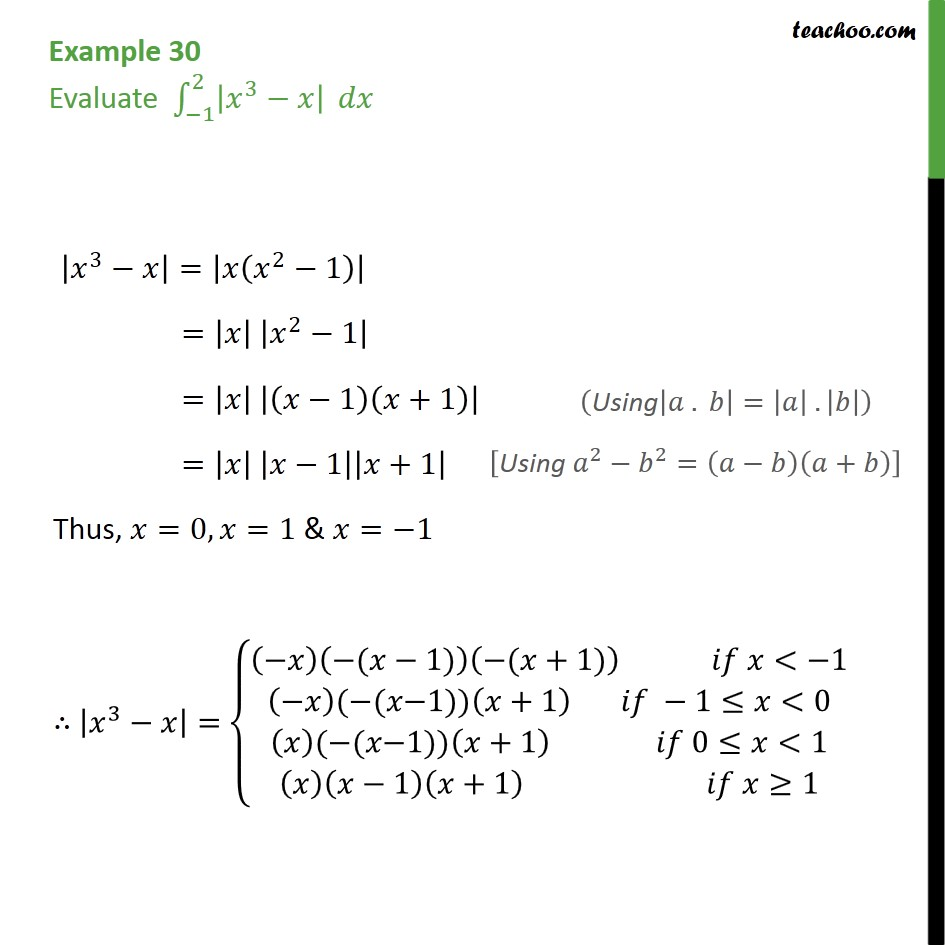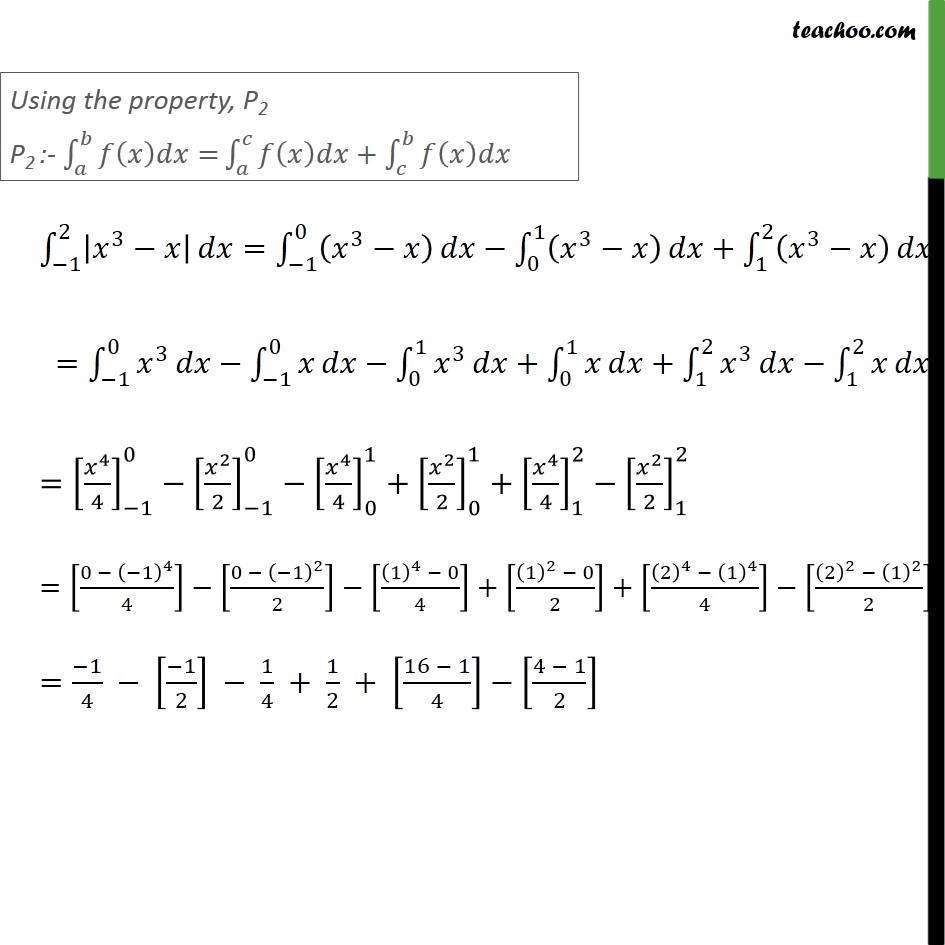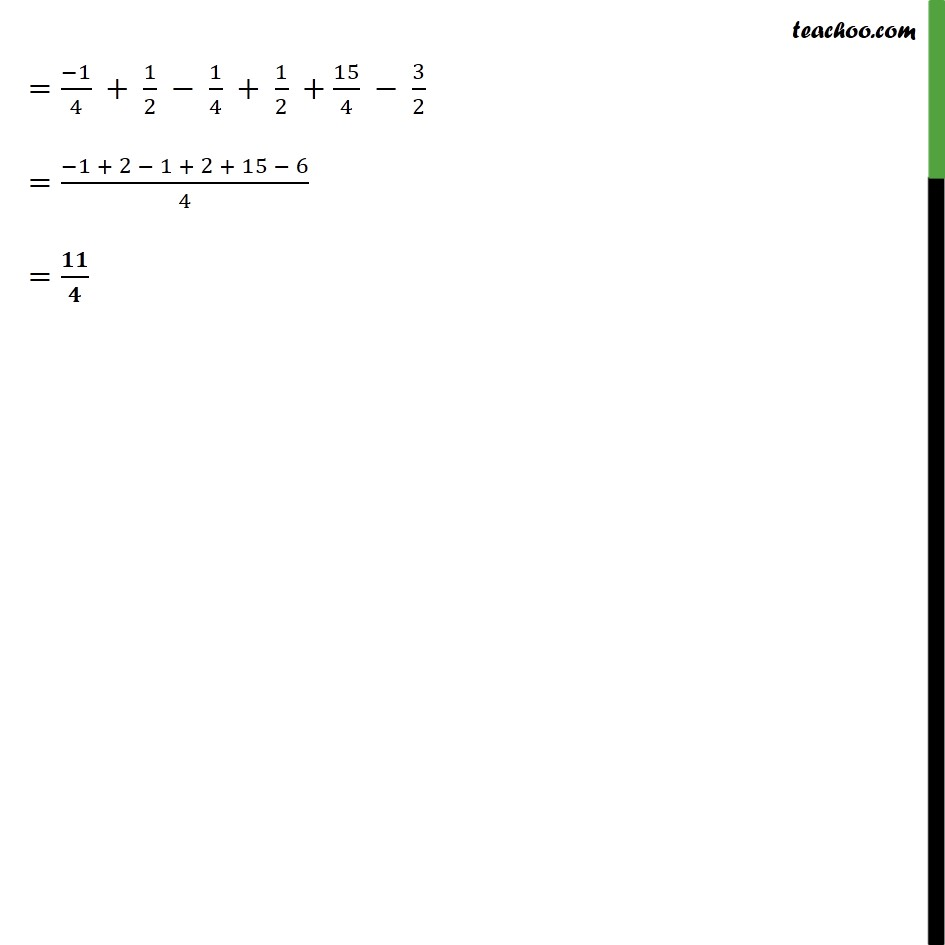Integration Full Chapter Explained - https://you.tube/Integration-Class-121. Chapter 7 Class 12 Integrals
2. Concept wise
3. Definite Integration by properties - P2

Transcript

Example 30 Evaluate 1 2 3 3 = 2 1 = 2 1 = 1 +1 = 1 +1 Thus, =0, =1 & = 1 3 = ( 1) ( +1) < 1 ( ( 1)) +1 1 <0 ( ( 1)) +1 0 <1 1 +1 1 3 = ( 3 ) < 1 ( 3 ) 1 <0 3 0 <1 3 1 1 2 3 = 1 0 3 0 1 3 + 1 2 3 = 1 0 3 1 0 0 1 3 + 0 1 + 1 2 3 1 2 = 4 4 1 0 2 2 1 0 4 4 0 1 + 2 2 0 1 + 4 4 1 2 2 2 1 2 = 0 1 4 4 0 1 2 2 1 4 0 4 + 1 2 0 2 + 2 4 1 4 4 2 2 1 2 2 = 1 4 1 2 1 4 + 1 2 + 16 1 4 4 1 2 = 1 4 + 1 2 1 4 + 1 2 + 15 4 3 2 = 1 + 2 1 + 2 + 15 6 4 =

Definite Integration by properties - P2